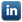## Exceltechie.com

Easiest way to learn excel on the planet|| Novice || Beginner || Intermediate || Advanced || Specialist || Expert ||
Types of Formula

 Financial Logical Informational Text Mathematical Statistical Lookup Reference Formula Errors

Alternatively you can also begin with ' + ' or ' - ' and start the Formulas.
Excel recognizes and converts the ' + 'and ' - ' to ' = 'Symbol when you hit enter
You may review the Cell Formula after you Hit enter to understand betterThe formula conversion does not happen when you use ' / ' or ' * ' arithmetic operators
You may alternative select a cell and Choose Fx from the Function tab to write formulas

Optional components in a formula are displayed within [] brackets
Eg:SUM( number1, [number 2], ... [number n] )
Formula and Results switching
Formulas ->Show Formulas - Switch between Formula and Results viewCTRL+`

Evaluate formula
Formulas -> Evaluate Formula - View how the formula is evaluated step by step